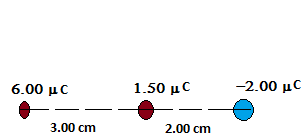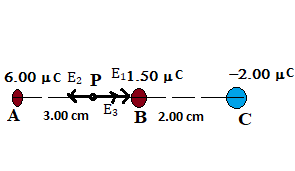# (a) Determine the electric field strength at a point 1.00 cm to the left of the middle charge...

## Question:

(a) Determine the electric field strength at a point 1.00 cm to the left of the middle charge shown in the figure below.

(b) If a charge of {eq}-3.80 \space \mu C {/eq} is placed at this point, what are the magnitude and direction of the force on it?## Electric Field

The electric field is qualitatively defined as the electrostatic force per unit charge when placed near a charged particle. The electric field due to point charge particle at a distance from the center of the particle is given by

{eq}\begin{align} E = \frac{kq}{r^2} \end{align} {/eq}

Where q is the charge on the particle and r is the distance from the center of the charge.

Data Given

Let us redraw the diagram showing the electric field at point P due to all charges,Now the net electric field at point P is given by

{eq}\begin{align} E = E_1 - E_2 + E_3 \end{align} {/eq}

{eq}\begin{align} E = \frac{kq_1}{AP^2} - \frac{kq_2}{BP^2} + \frac{kq_3}{CP^2} \end{align} {/eq}

{eq}\begin{align} E = \frac{9 \times 10^9 \ \rm Nm^2C^{-2} \times 6 \times 10^{-6} \ \rm C}{(0.02 \ \rm m)^2} - \frac{9 \times 10^9 \ \rm Nm^2C^{-2} \times 1.50 \times 10^{-6} \ \rm C}{(0.01 \ \rm m)^2} + \frac{9 \times 10^9 \ \rm Nm^2C^{-2} \times 2.0 \times 10^{-6} \ \rm C}{(0.03 \ \rm m)^2} \end{align} {/eq}

{eq}\begin{align} \color{blue}{\boxed{ \ E = 2.0 \times 10^7 \ \rm N/C \ }} \end{align} {/eq}

Part B)

• Charge placed at point P {eq}q = -3.80 \times 10^{-6} \ \rm C {/eq}

The force on the given charge is

{eq}\begin{align} F = qE \\ F = -3.80 \times 10^{-6} \ \rm C \times 2.0 \times 10^{7} \ \rm N/C \\ F = -76 \ \rm N \end{align} {/eq}

Now magnitude of the the force

{eq}\begin{align} \color{blue}{\boxed{ \ F = 76 \ \rm N \ }} \end{align} {/eq} and direction is towards the left.